# How to calculate and Solve for Shear Stress | Rock Mechanics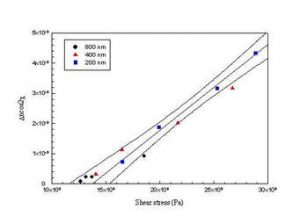The image above represents shear stress.

To compute for shear stress, two essential parameters are needed and these parameters are Shear Force (ΔS) and Area (ΔA).

The formula for calculating the shear stress:

τ = ΔS / ΔA

Where:

τ = Shear Stress
ΔS = Shear Force
ΔA = Area

Let’s solve an example;
Find the shear stress when the shear force is 16 with an area of 30.

This implies that;

ΔS = Shear Force = 16
ΔA = Area = 30

τ = ΔS / ΔA
τ = 16 / 30
τ = 0.53

Therefore, the shear stress is 0.53 Pa.

Calculating the Shear Force when the Shear Stress and Area is Given.

ΔS = τ x ΔA

Where;

ΔS = Shear Force
τ = Shear Stress
ΔA = Area

Let’s solve an example;
Find the shear force with a shear stress of 40 and an area of 12.

This implies that;

τ = Shear Stress = 40
ΔA = Area = 12

ΔS = τ x ΔA
ΔS = 40  x 12
ΔS = 480

Therefore, the Shear Force is 480.

Calculating the Area when the Shear Stress and Shear Force is Given.

ΔA = ΔS / τ

Where;

ΔA = Area
τ = Shear Stress
ΔS = Shear Force

Let’s solve an example;
Find the area with a shear stress of 480 and a shear force of 120.

This implies that;

τ = Shear Stress = 480
ΔS = Shear Force = 120

ΔA = ΔS / τ
ΔA = 480 / 120
ΔA = 4

Therefore, the area is 4.

Nickzom Calculator – The Calculator Encyclopedia is capable of calculating the shear stress.

To get the answer and workings of the shear stress using the Nickzom Calculator – The Calculator Encyclopedia. First, you need to obtain the app.

You can get this app via any of these means:

To get access to the professional version via web, you need to register and subscribe for NGN 1,500 per annum to have utter access to all functionalities.
You can also try the demo version via https://www.nickzom.org/calculator

Apple (Paid) – https://itunes.apple.com/us/app/nickzom-calculator/id1331162702?mt=8
Once, you have obtained the calculator encyclopedia app, proceed to the Calculator Map, then click on Geology under Add-on.Now, Click on Rock Mechanics under GeologyNow, Click on Shear Stress under Rock Mechanics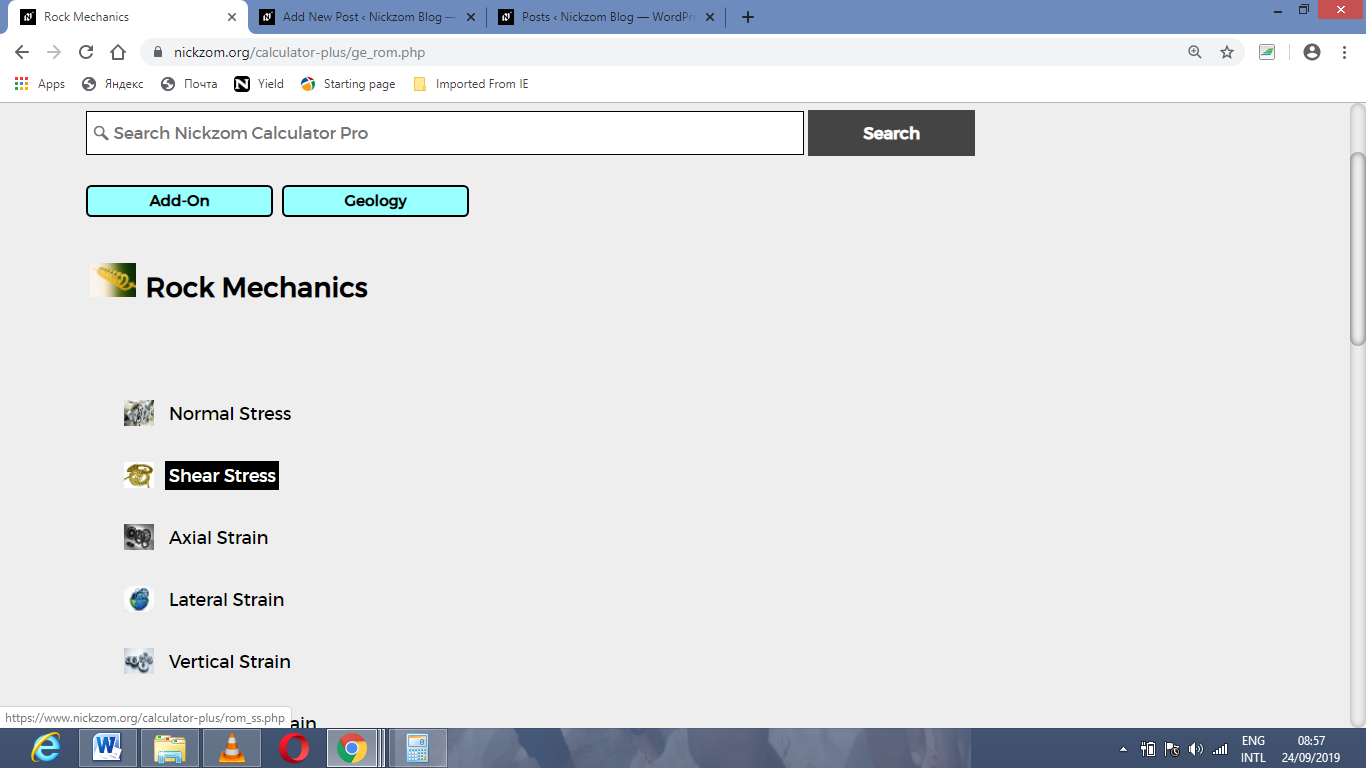The screenshot below displays the page or activity to enter your values, to get the answer for the shear stress according to the respective parameters which are the shear force (ΔS) and area (ΔA).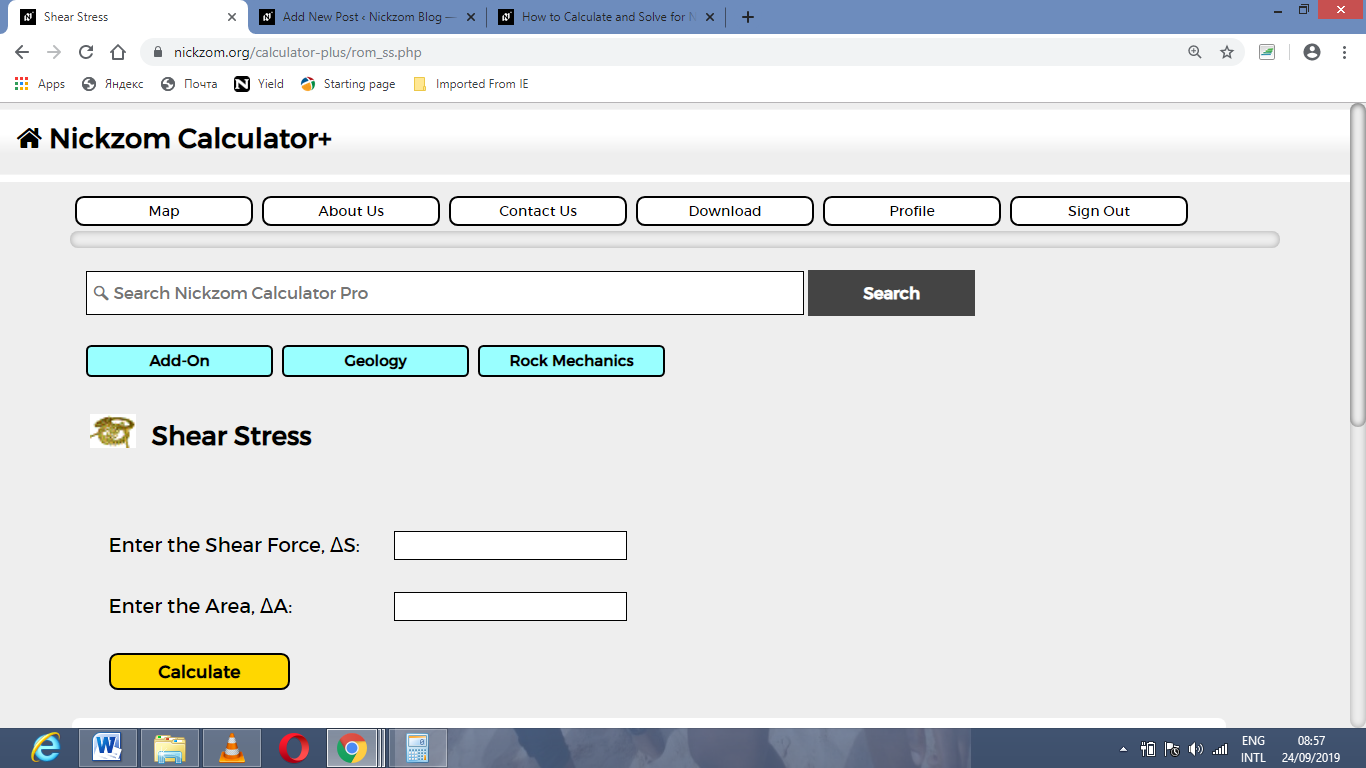Now, enter the values appropriately and accordingly for the parameters as required by the shear force (ΔS) is 16 and area (ΔA) is 30.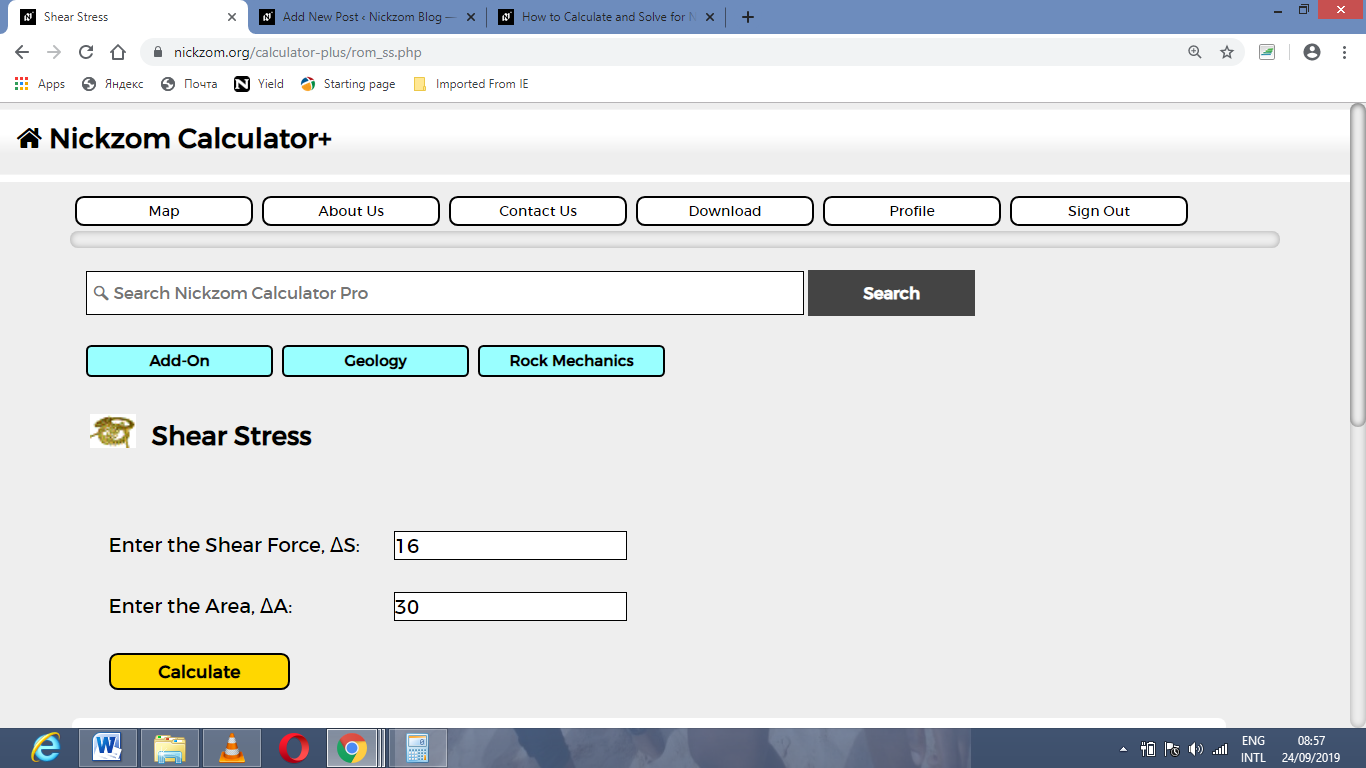Finally, Click on Calculate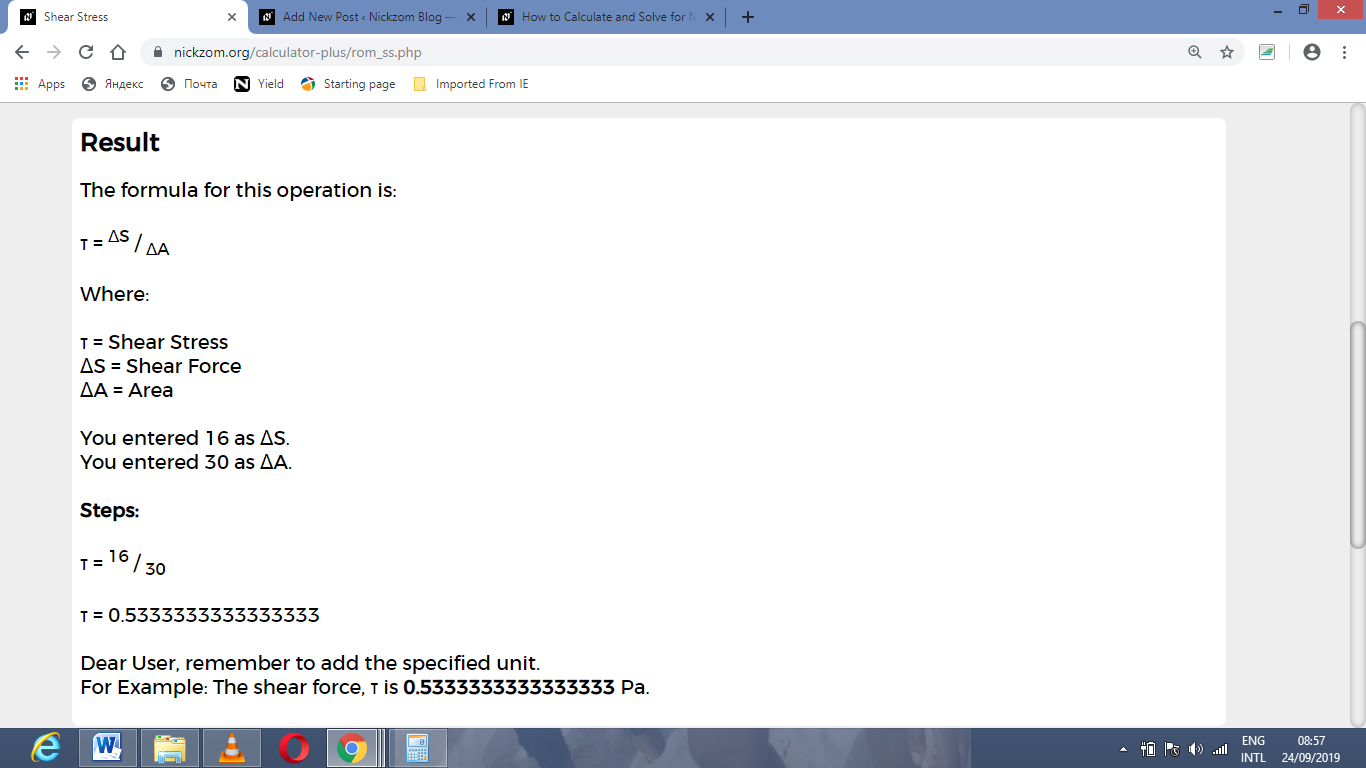As you can see from the screenshot above, Nickzom Calculator– The Calculator Encyclopedia solves for the shear stress and presents the formula, workings and steps too.